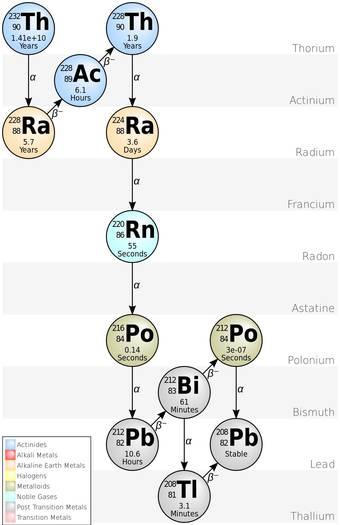#### Diagram showing the different stages of Thorium Decay. It does not include the more recently discovered nuclides that precede Thorium-232 in the chain.

Matters are made up of atoms and atoms are made up of a nucleus containing most of the mass, and of electrons. The nucleus is a bound state of protons and neutrons held together by the strong nuclear force and the charge of the nucleus, which determines the chemical properties of the atom, is equal to the number of protons in it. Sometimes neutrons and protons are collectively referred to as ‘nucleons’.

The presence of neutrons in the nucleus is essential to make the nucleus stable and a nucleus needs an approximately equal number of protons and neutrons to be stable. But this is not always true; high-mass nuclei need more neutrons than protons to be stable. This disparity between neutron and proton gives to a new form of atoms, called isotopes.

Nuclei of the same charge but with a different number of neutrons are called isotopes. So, each isotope of a given element has a different mass number.

For example, carbon-12, carbon-13, and carbon-14 are three naturally occurring isotopes of the element carbon with mass numbers 12, 13, and 14, respectively. (There exists one more isotope of carbon- carbon-11, which is not a naturally occurring isotope).

Among the isotopes of an element, some are stable and some are unstable where the unstable isotope decay until a stable configuration is reached (in one or more steps) by losing energy in the form of radiation. The decay process is called radioactive decay. There are three most common types of decay as alpha decay, beta decay, and gamma decay. Each decay emits alpha particle which is helium-4 nuclei, beta particle which is electrons, and gamma rays which is electromagnetic radiation like X-ray except much higher in energy and even more dangerous to living systems, respectively. The decay process that will be followed by an element depends on factors like the number of protons, neutrons, and how much heavier the element is.

The radioactive decay is governed by relation,

N(t) = N(0)e-t/𝛕

where N(t) is the number of atoms at any time, N(0) is the number of atoms at time (t) =0, 𝛕 is the average decay time given by T1/2 = 𝛕 ln 2, T1/2 is the half-life.

Alpha Decay

Most often, atoms with massive nuclei that have too large a proton to neutron ratio undergo alpha decay producing an alpha particle which is, as earlier said, 4He nucleus. An alpha particle, with its two protons and two neutrons, is a very stable configuration of particles. During this decay process, the mass number of the parent nucleus will be reduced by four and the atomic number will be reduced by two.

The reaction is,

NXZ      --------(alpha decay)---------       N-2XZ-4     +      2He

Where N is the atomic number and Z is the mass number.

Due to the change in atomic number alpha decay give rise to a different daughter nucleus and so have different chemical properties than the parent nucleus.

For example,

84Po210   ---------(alpha decay)-------------  82Pb206   +   2He4

Remember that the atomic number is the number of protons in an atom. So the number of protons in Po is 84 and the number of neutrons is 126. This gives the ratio of the number of protons to the number of neutrons in Po (=84/126) to be 0.667. Now the daughter nucleus, the number of protons is 82 and the number of neutrons 124 giving the ratio of the number of protons to neutron (=82/124) 0.661. Notice that this small change in the ratio of the number of protons to neutrons is enough to make Po stable.

Some atom can go multiple decays until it comes to a stable state where they can also involve in other decay like beta decay.

For instance, 92U238  will eventually be converted into 82Pb206 which is stable by multiple alpha and beta decay.

You may look into National Nuclear Data Center for decay products of different elements.

In the alpha decay of a nucleus, the change in binding energy appears as the kinetic energy of the alpha particle and the daughter nucleus. Because this energy must be shared between these two particles, and because the alpha particle and daughter nucleus must have equal and opposite momenta, the emitted alpha particle, and the recoiling nucleus will each have well-defined energy after the decay. Because of its smaller mass, most of the kinetic energy goes to the alpha particle.